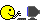# Decimal comparison oddity: sharing how I solved it

Hello,
While coding tests for a hobby project of mine, I stumbled upon something odd when directly comparing `Decimal`s – which is a bad idea but it’s inevitable when doing `assert` equal in tests with maps that contain `Decimal`s.

``````iex> {:ok, d1} = Decimal.parse("00020.400")
{:ok, #Decimal<20.400>}
iex> {:ok, d2} = Decimal.parse("00020.4")
{:ok, #Decimal<20.4>}
iex> Decimal.cmp(d1, d2)
:eq
iex> > d1 == d2
false
``````

The last part made my tests fail. What further surprised me is that there is a way to equalize `Decimal`s that have to parse such zero-padded data: Decimal.reduce.

``````iex> {:ok, d1} = Decimal.parse("00020.400"); d1 = Decimal.reduce(d1)
{:ok, #Decimal<20.4>}
iex> {:ok, d1} = Decimal.parse("00020.4"); d2 = Decimal.reduce(d2)
{:ok, #Decimal<20.4>}
iex> d1 == d2
true
``````

Now the tests succeed.

Maybe this will help you one day.(Alternatively, you could just do `String.trim(your_string, "0")` before parsing, which will rid you of all zeroes both in front and at the back.)

4 Likes

`==/2` does structural compasions and not logical. Therefore you shouldn‘t use it for structs, where different values in the structs‘ fields are still considered the same value, which is true for decimals (different number ot trailing 0‘s) but also for some core structs like datetimes. Use functions of those structs to compare them instead of the equals operator.

3 Likes

It’s just that in this case I was doing something like `assert parse_struct_from_textual_input(...) == expected_hardcoded_struct` and didn’t stop to think further (which is of course my mistake). I should probably just write an `equals(a, b)` function in the struct modules and use that instead of a `==` assert.